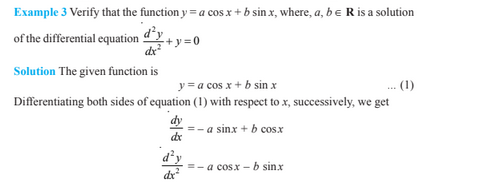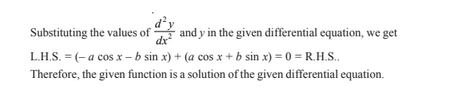# Differential Equations For Class 12

Differential equations class 12  helps students to learn how to differentiate a function “f” with respect to an independent variable. A differential equation is of the form dy/dx= g(x), where y= f(x). These equations arise in a variety of applications, may it be in Physics, Chemistry, Biology, Anthropology, Geology, Economics etc. In differential equations class 12, we are going to study the basic concepts related to differential equations in detail.

## Differential Equations Class 12 Topics

The topics and sub-topics covered in differential equations class 12 are:

• Introduction
• Basic Concepts
• Order of a differential equation
• Degree of a differential equation
• General and Particular Solutions of a Differential Equation
• Formation of a Differential Equation whose General Solution is given
• Procedure to form a differential equation that will represent a given
family of curves
• Methods of Solving First Order, First Degree Differential Equations
• Differential equations with variables separable
• Homogeneous differential equations
• Linear differential equations

## Differential Equations Class 12 Notes

Here, the basic concepts included in the differential equations class 12 are given with examples.

Order of differential equation

The highest order of the derivative present in the dependent variable with respect to the independent variable in the given differential equation.

$\left ( \frac{d^{3}y}{dx^{2}} \right ) + x\left ( \frac{dy}{dx} \right )^{4} = 0$

In the example given above, the order of the differential equation is 3.

General and Particular Solution of a Differential Equation-

The solution which contains arbitrary constants is called the general solution of the differential equation.

Whereas the solution free from arbitrary constants obtained from the general solution by giving particular values to the arbitrary constants is called a particular solution of the differential equation.

Formation of a Differential Equation whose General Solution is given

The order of a differential equation representing a family of curves is the same as the number of arbitrary constants present in the equation corresponding to the family of curves.

Methods of Solving First Order, First Degree Differential Equations

There are three methods to solve the first-order, first-degree differential equations. They are:

• Differential equations with variables separable
• Homogeneous differential equations
• Linear differential equations

### Differential Equations Class 12 Example ProblemsStau tuned with BYJU’S – The Learning App and also watch personalized videos to learn with ease.